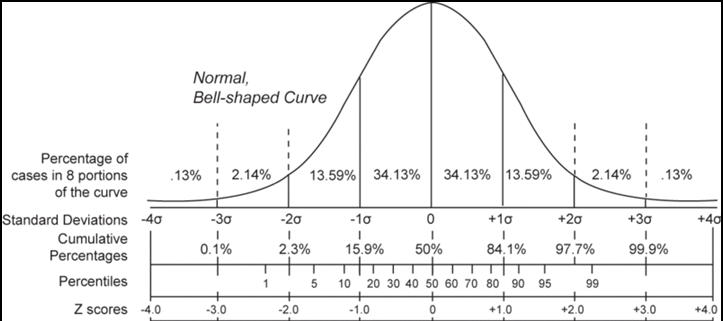# Exploring the normal distribution

The normal distribution has two parameters: $$\mu$$ and $$\sigma$$ and is usually indicated as $$N(\mu,\sigma)$$. Is this application you can explore several features of the normal distribution. For instance, given a value of k, the probabiliy of observing a value within the interval $$\mu \pm k \times \sigma$$ is always the same for any normal, independently of the parameter values. In when $$k=1.96$$ that probability is 0.95.
The area under two values of X indicates the probability of obtaining values within this two values. For example, if the area is equal to 0.69, this meants tha if we observe a subject from this population its measured value of X will be within this interval in the 69% of the cases.
A sample of individuals will show a distribution (histogram) that is in accordance with the normal curve that represents the behaviour of this variable in the population.

### Parameters

In a normal distribution $$N(\mu,\sigma)$$, the parameter $$\mu$$ defines the position of the distribution. This distribution is symmetric with respect $$\mu$$.That is: $$P(X>\mu)=P(X<\mu)=0.5$$. The parameter $$\sigma$$ indicates the amplitude of the possible values of X. The arrow in the graphic show the value of this parameter fixed in the parameters panel. For a fixed value of $$k$$, the probability of the interval $$\mu \pm k \times \sigma$$ is always the same, independently of the parameter values. The probability of obtaining a value offside the interval $$\mu \pm 3 \times \sigma$$ is almost 0.

$$P(\mu - k \times \sigma < X < \mu + k \times \sigma )$$

If $$X$$ is a $$N(\mu,\sigma), then:$$

The probability $$P(X<x)$$ is obtained with the instruction:

### pnorm(x,mu,sigma)

The probability $$P(X>x)$$ is obtained with the instruction:

### 1-pnorm(x,mu,sigma)

The probability of an interval $$P(a<X<b)$$ is obtained with the instruction:

### pnorm(b,mu,sigma)-pnorm(a,mu,sigma)

The value of $$a$$ that fulfils $$P(X<a)=\alpha$$ is obtained with the instruction:

### Computing probabilities

If $$X$$ is a normal distribution $$N(\mu,\sigma)$$, the the variable: $$Z=\frac{X-\mu}{\sigma} \rightarrow N(0,1)$$ The $$N(0,1)$$ is called normal standard and is denoted by $$Z$$
If $$X$$ is a normal distribution $$N(\mu,\sigma)$$, then: $$P(X\le x)=P \left( Z \le \frac{x-\mu}{\sigma}\right)$$

#### Example

Let's $$X$$ be a normal distribution $$N(100,3)$$
Then: $$P(X\le 102)=P \left( Z \le \frac{102-100}{3}\right) = P(Z \le 0.67) = 0.7475$$ In R it is equivalent to compute:

### Computing quantiles

The value $$x_\alpha$$ that fulfils the equation $$P(X \le x_\alpha)=\alpha$$ is called quantile $$\alpha$$ . Using the standard normal: $$P(X \le x_\alpha)=P \left( Z \le \frac{x_\alpha-\mu}{\sigma} \right)=\alpha$$ Then $$z_\alpha=\frac{x_\alpha-\mu}{\sigma} \rightarrow x_\alpha=\mu+z_\alpha \sigma$$

#### Example

Let's $$X$$ be a normal distribution $$N(50,2)$$
The quantile 0.92 is computed as qnorm(0.92,50,2), which gives a result of 52.81014 The quantile 0.92 for the standard normal, i.e. $$z_{0.92}$$ is obtained as  qnorm(0.92) which gives 1.405072 You can check that: 50+1.405072*2=52.81014### Z-scores

The z-score of an observed value $$x$$ of a $$N(\mu,\sigma)$$ is: $$z=\frac{x-\mu}{\sigma}$$
If $$\bar X$$ is the mean of a sample, and $$s$$ is the correspondiong standard deviation, then the z-score is obtained as: $$z=\frac{x-\bar X}{s}$$

### Interpretation

The z-score indicates how many times the observed value $$x$$ is above (or below in case it is negative) the mean in units of standard deviations.

As z-scores are distributed as a $$N(0,1)$$, its value can be read in terms of this distribution. For example, supose a man that has a weight of 90.3 kg. In the population, we have a mean of 80.3kg. with a standard deviation of 5.3kg. In tha case, the z-socre for this man is: $$z =\frac{90.3-80.3}{5.3}=1.89$$ In a $$N(0,1)$$ the $$P(Z<1.89)=0.97$$ this means this man has a percentile of weigth of 97 and that few people on this population will have a higher weight.

Z-scores allow comparing variables with different measurement units. For example, if an individual has a z-score of 2.1 for weight and 0.3 for height, this indicates that is close to the mean in height but well above the mean in weight!

### Sample statistics

(c) Albert Sorribas, Ester Vilaprinyo, Rui Alves, Montserrat Rue
Biomodels Group
University of Lleida - Institute of Biomedical Research (IRBLleida)